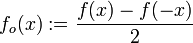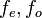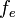# Odd part

## Definition

Suppose$f$ is a function whose domain is a subset of the reals that is symmetric about 0, i.e., for every$x$ in the domain of$f$,$-x$ is also in the domain of$f$. Then, the odd part of$f$, sometimes denoted$f_o$ or$f_{\operatorname{odd}}$ is defined as a function with the same domain, and with the definition:$\! f_o(x) := \frac{f(x) - f(-x)}{2}$

Equivalently, it is the only possible choice of odd function in a decomposition of$f$ of the form:$\! f(x) = f_e(x) + f_o(x)$

with$f_e, f_o$ both having the same domain as$f$, and with$f_e$ an even function and$f_o$ an odd function. The other part,$f_e$, is the even part of$f$.

## Facts

### Effect on even and odd functions

• The odd part of an even function is the zero function on the same domain.
• The odd part of an odd function is the same function.

### Properties preserved on taking the odd part

Function property Explanation for why taking the odd part preserves this property
continuous function odd part of continuous function is continuous
differentiable function odd part of differentiable function is differentiable
periodic function odd part of periodic function is periodic
infinitely differentiable function odd part of infinitely differentiable function is infinitely differentiable
analytic function odd part of analytic function is analytic

### Effect of operators on odd part

Operator Effect on odd part (short version) Effect on odd part (in symbols) Proof
pointwise sum the odd part of a sum of functions is the sum of the odd parts of each function Failed to parse (syntax error): (f + g)_o = f_o + g[[odd part operator is linear]] |} ==Particular cases== {| class="sortable" border="1" ! Function !! Domain !! Odd part |- | [[polynomial function]] || all reals || sum of all the monomials of odd degree in the polynomial |- | [[exponential function]] [itex]e^x all reals hyperbolic sine function$\sinh$